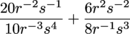# TSI Math: Using Every Trick in the Box to Simplify an Expression

Simplify the following expression.Intermediate Algebra and Functions Rational and Exponential Expressions, Equations, and Functions Mathematics and Statistics Assessment Rational and Exponential Expressions, Equations, and Functions Product Type TSI TSI Mathematics and Statistics AssessmentTSI MathTSI Mathematics TSI Math Intermediate Algebra and Functions TSI Mathematics Intermediate Algebra and Functions Test Prep TSI

### Transcript

00:23

than they are in watching us create scale models of

00:26

famous landmarks using pasta weird we're so good at that

00:29

00:31

many ways to begin simplifying this gnarly crazy exponential expression

00:36

but well here we're going to eliminate negative exponents first

00:39

to make the exponents positive move the variable to the

00:43

opposite location in the fraction variables in the numerator go

00:47

to the denominator and variables in the denominator go to

00:50

the numerator see how we do this here So we've

00:52

got well that we're starting with the twenty are the

00:55

negative too and then ethan and stuff and then we're

00:58

going to do is just move everything here flip flop

01:01

so we've got this ugly beast right here so we

01:04

simplified by multiplying variable terms with the same bases together

01:07

When this happens the exponents are added so that gets

01:11

us What is that twenty r cubed And here And

01:14

then in six r squared plus once we add the

01:16

two in the one there when we have in our

01:17

times and our down here we've gotten as to the

01:20

first honest to the force it's nice They put the

01:23

s to the first there on the exam They may

01:24

not be so kind so that gets us as to

01:27

the fifth here on the bottom And then we got

01:29

as to the third And as to the second there's

01:31

what's going on adam And that consists s to the

01:33

fifth All right so this is way simpler here Twenty

01:35

r cubed over ten r squared as to the fifth

01:38

plus six r cubed over eight s to the fifth

01:40

So next up when dividing factors with like bases like

01:44

you know the r's and the first fraction there subtract

01:47

the exponents so we can actually do that that's legal

01:49

even in even in texas So we've got twenty are

01:52

cube there and we're just going to subtract the two

01:55

from down here to get rid of it So what

01:58

does that make it All right so then we've got

01:59

twenty art of the first Well over ten as to

02:02

the fifth and then six r q P o radius

02:04

Okay well so what else can we do here tio

02:08

Simplify things Well finally we can simply the coefficients of

02:12

each fraction is if they didn't have dedicated variable followers

02:15

02:18

zeros there Twenty over ten becomes too over one And

02:21

just two And the six over eight There becomes three

02:23

force So we got two r over us to the

02:26

fifth plus three r cubed over for s to the

02:29

fifth Okay so that's about as simple as we can

02:32

get but we're not done The simplified expression is not

02:35

the same as this Eight are over us and so

02:39

on Equation on d there which is one big hint

02:42

The fractions should be added Multiply the first fraction by

02:45

four over four to create a common denominator of four

02:49

ass to the fifth See how clever that is So

02:51

we can go for over four times as so we

02:53

get eight And then plus the three are there over

02:57

03:00

of a luxury around here So what does that Give

03:02

us well added new rangers Crossed top and simplest expression

03:04

is b eight are plus three r cubed over for

03:07

ass to the fifth Okay we're done We're shmoop